# How to Get the Key with the Maximum Value in Python

Sometimes, when we’re counting occurrences of an item, we need to obtain the item with the highest frequency.

Another way to express this is: how can we get the key with the maximum value in a dictionary?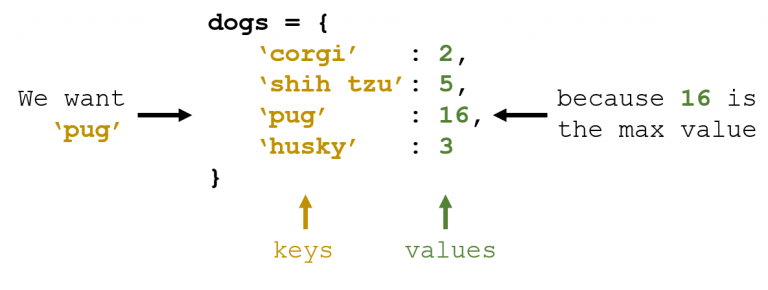Well. There are many ways to do this:

Given a dictionary structured as follows, we’ll walk through six methods to obtain the key with the maximum value.

``````d = {
'corgi': 2,
'shih tzu': 5,
'pug': 16,
'husky': 3
}
``````

## Method 1

To understand this method, it’s important to know that you can obtain the keys and values of a dictionary using `d.keys()` and `d.values()`. They will return an iterable list of keys and values, respectively.

`arr.index(elem)` will provide the index of `elem` in array `arr`.

This method involves converting all the keys and values into separate lists, finding the maximum value, obtaining its index, and returning the corresponding value in the keys list.

``````def method1():
k = list(d.keys())
v = list(d.values())
return k[v.index(max(v))]
``````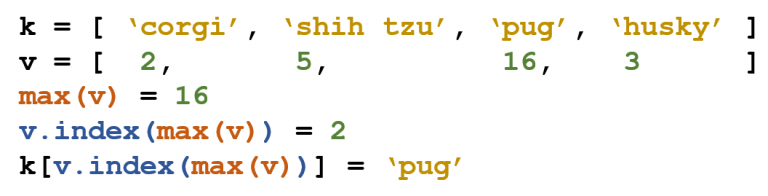## Method 2

Similar to `d.keys()` and `d.values()`, `d.items()` will return both the keys and values in a list of tuples.

Additionally, `max(d)` will provide us with the maximum key of dictionary `d`.

In this method, we reverse the original dictionary, call `max()` to get the maximum key in the new dictionary, and then look up the value associated with that key.

``````def method2():
d_new = { v: k for k,v in d.items() }
return d_new[max(d_new)]
``````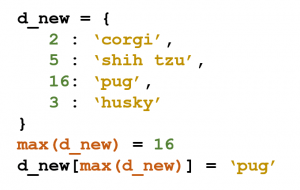## Method 3

This third method makes use of `lambda` functions and the `filter()` method.

Similar to how we can use `def` to define a function, `lambda` can be used to define an inline, anonymous function.

`filter()` allows us to select only elements in a list that follow some condition. We use a `lambda` function to specify this condition. As an example, the following `lambda` function will return only the objects whose first element is equal to `5`.

``````lambda t: t == 5
``````

Be sure to read up on `lambda` functions.

This method uses the `lambda` function and `filter()` to retrieve only the sets of key-value pairs whose value is equivalent to the maximum value in the entire dictionary. It is then converted to a `list` and indexed to obtain just the key.

``````def method3():
return list(filter(lambda t: t == max(d.values()), d.items()))
``````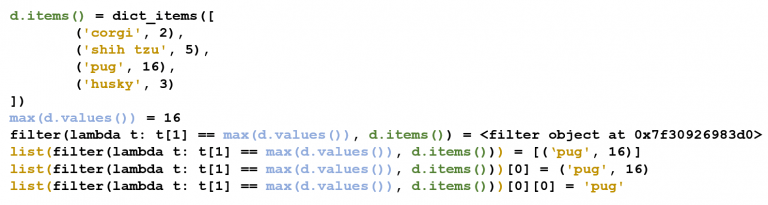## Method 4

In this method, we utilize the `max()` function again to easily obtain the maximum of any given collection.

However, this time, we use the `key` parameter, which defines the basis of comparison.

In this case, we are evaluating the maximum in `d.items()` based on the second element, or the first index. The second element in each `d.items()` object is the value in the dictionary. As a result, we are comparing the value field, giving us the maximum value.

To do this, we will use the built-in `operator` module. You can read up on `operators` and `itemgetters`.

``````import operator
def method4():
return max(d.items(), key = operator.itemgetter(1))
``````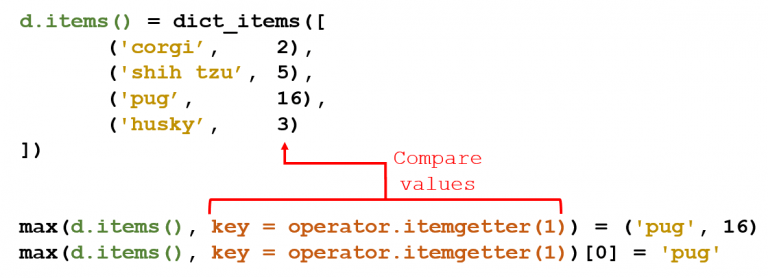## Method 5

The fifth and sixth methods are essentially the same as the fourth, but they take a different route to define the key.

In this method, we use the built-in `get` method for dictionaries, which returns the value for each key in the dictionary.

``````def method5():
return max(d, key = d.get)
``````

## Method 6

We can create the same functionality using a `lambda` function.

Each key will simply be outputting its respective value in the dictionary. This is the same idea as the previous method.

``````def method6():
return max(d, key = lambda k: d[k])
``````

## Which Method is the Fastest?

There are all these different methods, but which one should you use?

I used Python’s `timeit` module to time each method separately. This module allows us to record the time it takes to execute any number of lines in a given program one million times.

I created the dictionary in `test_dict` and surrounded it in quotes. timeit will only execute lines inside quotes ("…execute me…") as shown below. To execute multiple lines, we can surround the lines in three quotes ("""…execute me…""").

``````test_dict = "d = {'corgi': 2, 'pug': 5, 'shih tzu': 16, 'husky': 3}"
​
method1 = """
v = list(d.values())
k = list(d.keys())
k[v.index(max(v))]
"""
method2 = """
d_new = { v: k for k,v in d.items() }
d_new[max(d_new)]
"""
method3 = """
list(filter(lambda t: t == max(d.values()), d.items()))
"""
method4 = """
import operator
max(d.items(), key = operator.itemgetter(1))
"""
method5 = "max(d, key = d.get)"
method6 = "max(d, key = lambda k: d[k])"
``````

Since `test_dict` is input as the setup parameter, the time it takes to run `test_dict` will not be included in our timing.

Only the code inside `method#` (`method1`, `method2`, etc.) will be run, as indicated by the `stmt` (statement) parameter.

``````import timeit
# 4.646706566985813
print(timeit.timeit(stmt = method1, setup = test_dict))
# 4.319815654002014
print(timeit.timeit(stmt = method2, setup = test_dict))
# 11.278023117003613
print(timeit.timeit(stmt = method3, setup = test_dict))
# 5.527265636992524
print(timeit.timeit(stmt = method4, setup = test_dict))
# 2.376368289013044
print(timeit.timeit(stmt = method5, setup = test_dict))
# 3.923244642006466
print(timeit.timeit(stmt = method6, setup = test_dict))
``````

Over one million iterations, Method 5 runs faster than every other method, with Method 6 coming in second.

``````def method5():
return max(d, key = d.get)
``````

This is the fastest and probably the cleanest way to obtain the key with the maximum value in a dictionary.